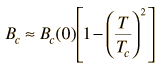# Critical Magnetic Field and Critical Current

It is the nature of superconductors to exclude magnetic fields (Meissner effect) so long as the applied field does not exceed their critical magnetic field. This critical magnetic field is tabulated for 0K and decreases from that magnitude with increasing temperature, reaching zero at the critical temperature for superconductivity. If the critical magnetic field is exceeded, the material makes a transition out of superconductivity to normal conductivity. The critical magnetic field at any temperature below the critical temperature is given by the relationshipFor a superconductor with critical temperature Tc = K

and a critical magnetic field at zero Kelvin of Bc(0) = T,

then at a temperature of T = K

the critical magnetic field is Bc = T.

The existence of a critical magnetic field implies the existence of a maximum current in a wire of the superconducting material because the current itself generates a magnetic field according to Ampere's law.

For a wire of radius cm = micrometers (microns)

the maximum current is Ic = Amperes.

 Illustrate mixed state Examples
 Table of critical fields and temperatures
Index

Superconductivity concepts

Reference Rohlf,Ch 15

 HyperPhysics***** Condensed Matter R Nave
Go Back